Tablecloth

I embroider tablecloth in 20 days but if I embroider 3/4 hours a day I have embroider it for 15 days. How long do I embroider a day?

Result

x =  1 h

Solution:

3/4*20 = 15x

60x = 60

x = 1

Calculated by our simple equation calculator.

Leave us a comment of example and its solution (i.e. if it is still somewhat unclear...):Be the first to comment!To solve this example are needed these knowledge from mathematics:

Do you have a linear equation or system of equations and looking for its solution? Or do you have quadratic equation?

Next similar examples:

1. Chocolate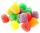I eat 24 chocolate in 10 days. How many chocolate I eat in 15 days at the same pace?
2. Three paintersOne painter would have painted the fence for 15 hours, the second in 12 hours. The plot had to be painted in four hours, so they called the third one, and all worked together. For what time would the third painter paint fence the fence alone?
3. Painting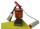Jožko with Fero painted the bench for four hours. How many hours would take work if four friends help him?
4. Hectoliters of waterThe pool has a total of 126 hectoliters of water. The first pump draws 2.1 liters of water per second. A second pump pumps 3.5 liters of water per second. How long will it take both pumps to drain four-fifths of the water at the same time?
5. Pumps A and BPump A fill the tank for 12 minutes, pump B for 24 minutes. How long will take to fill the tank if he is only three minutes works A and then both pumps A and B?
6. QuizTested student answered correctly on a 4/ 8 questions. Wrong answers was 16. How many questions answered?
7. 1.5 divided1.5 divided by 1 = w divided by 4
8. Book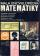To number pages of thick book was used 4201 digits. How many pages has this book?
9. Two ages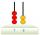Gabrielle is 9 years younger than Mikhail. The sum of their ages is 87. What is Mikhail's age?
10. After 16 years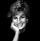After 16 years will Dana be five times older as she was four years ago. After how many years will Dana celebrate her 16th birthday?
11. BicycleThe bicycle pedal gear has 36 teeth, the rear gear wheel has 10 teeth. How many times turns rear wheel, when pedal wheel turns 120x?
12. Simple equationSolve for x: 3(x + 2) = x - 18
13. CakesGrandmother baked cakes. Half of its was poppy, quarter with plum jam and 16 cheesecakes. How many cakes she baked in total?
14. NormThree workers planted 3555 seedlings of tomatoes in one dey. First worked at the standard norm, the second planted 120 seedlings more and the third 135 seedlings more than the first worker. How many seedlings were standard norm?
15. Six yearsIn six years Jan will be twice as old as he was six years ago. How old is he?
16. Unknown numberIdentify unknown number which 1/5 is 40 greater than one tenth of that number.
17. Equation 29Solve next equation: 2 ( 2x + 3 ) = 8 ( 1 - x) -5 ( x -2 )